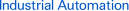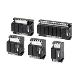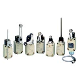Page top# FAQ02990 of Limit Switches FAQ

FAQ No. FAQ02990

Question

How do you calculate the operating force (OF) when changing the length of an adjustable roller lever in Limit Switches?

﻿

Use the following equation:

OF' = (OF x R)*/R'

*(OF x R): Fixed torque

Where OF is operating force, R is roller lever length, R' is the desired roller lever length, and OF' is operating force for the desired roller lever length.

For example, using the WLH12 Limit Switch with a roller lever length of 89 mm will result in the following OF':

(9.81 N x 38 mm)*/89 mm = Approx. 4.19N

*9.81 N: Standard OF
38 mm: Lever length for standard OF

In the above equation, the standard OF for a WLH12 with a roller lever length of 38 mm is 9.81 N (the OF listed in the datasheet), so the fixed torque is calculated as OF x R.

Dividing this by R' (89 mm in this example), which is the desired roller lever length, gives us the OF', which is the operating force for a Limit Switch with the desired roller lever length.

## Recommended Products

###61F-G[]NImproved Design for a More Lightweight Construction and Reduced Standby Power Consumption.

###ZBest-selling Basic Switch Boasting High Precision and Wide Variety

###WL, WLMWide Range of Two-circuit Switches; Select One for the Operating Environment/Application